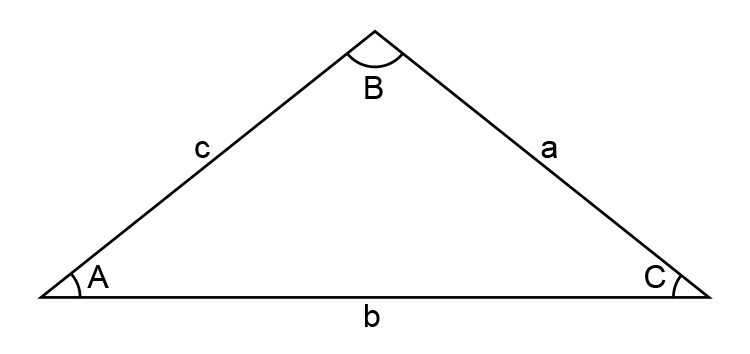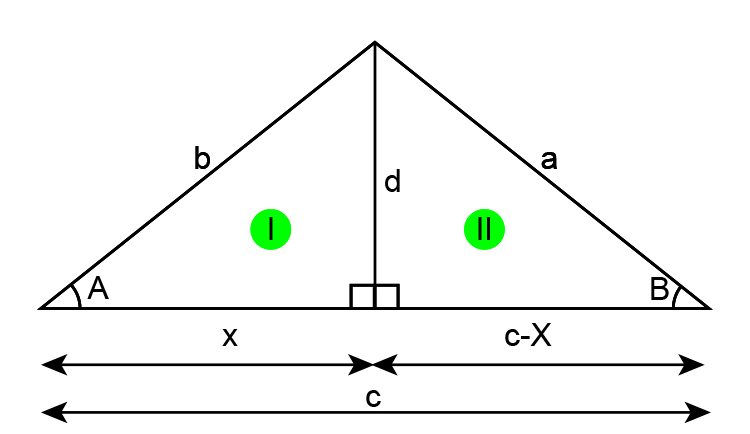# Where does the cosine rule come from?

For the mathematical puritans out there the cosine rule derives from the following:

(you really don’t need to know this)Find “a” KNOWING the angle A and length b and c.

Redraw the diagramPythagoras tells us that in triangle I ,  x^2+d^2=b^2 ................  equation 1

Trigonometry tells us that cosA=x/b

Therefore  x=bcosA ………… equation 2

Pythagoras also tells us that  (c-x)^2+d^2=a^2  …………… equation 3

Multiplying equation 3 out we get:  (c-x)(c-x)+d^2=a^2

c^2-cx-cx+x^2+d^2=a^2

c^2-2cx+x^2+d^2=a^2 ……………. equation 4

Substitute equation 1 into equation 4 we get:

c^2-2cx+b^2=a^2

Replacing x with equation 2 we get:

c^2-2c(bcosA)+b^2=a^2

Rearrange slightly

a^2=b^2+c^2-2cb\cosA

But again we emphasise that you really don’t need this.